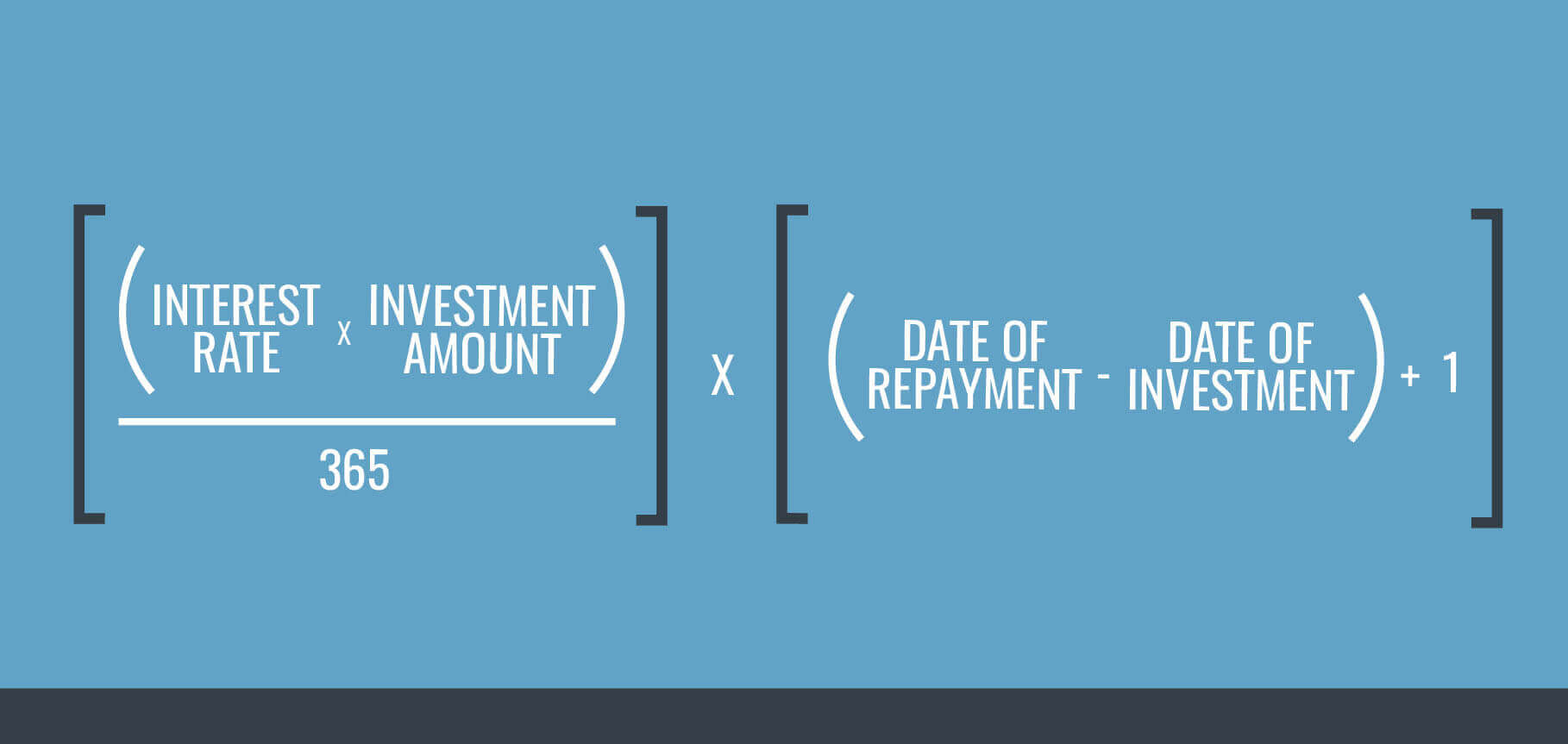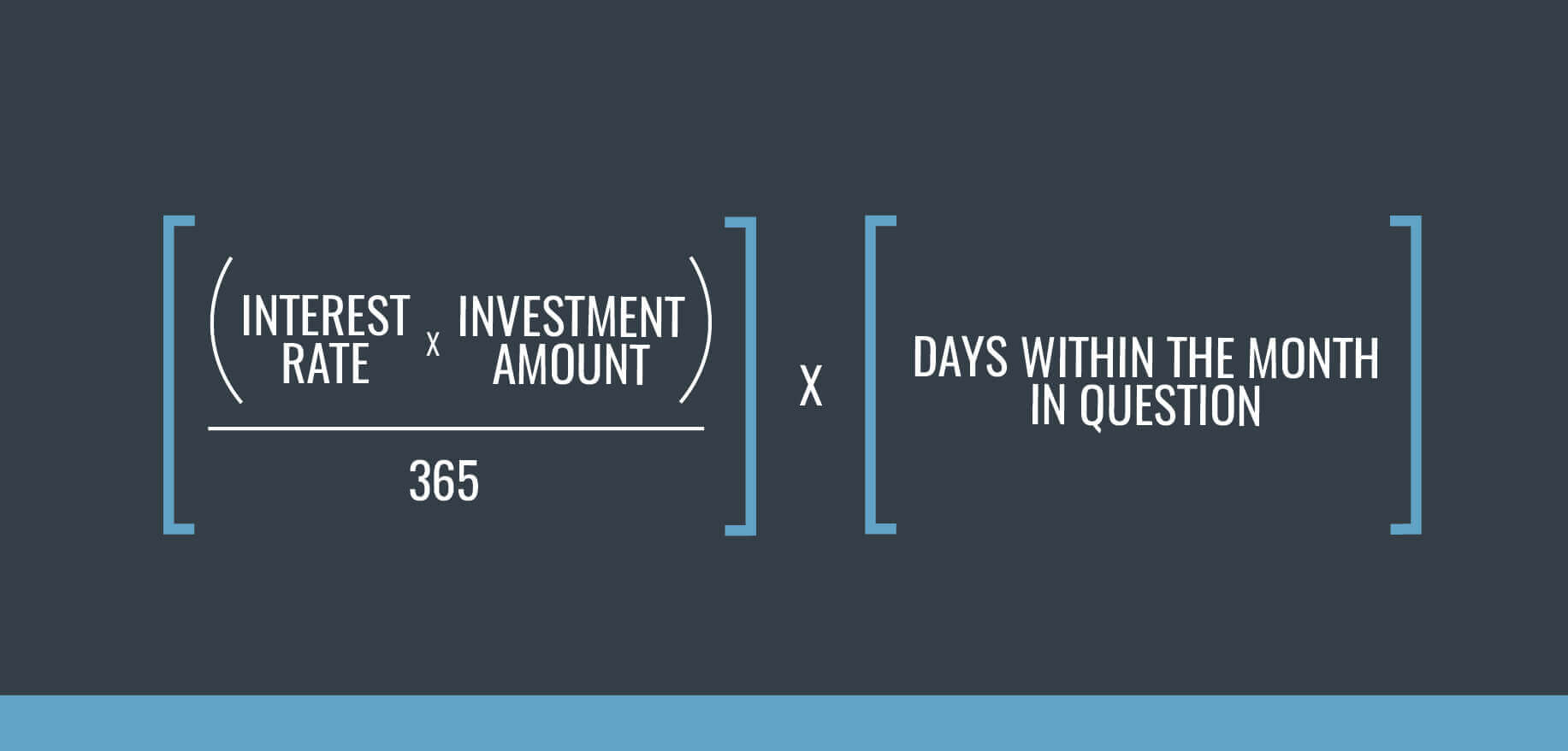<img height="1" width="1" src="https://www.facebook.com/tr?id=651326152448638&amp;ev=PageView &amp;noscript=1">

# Here's How GROUNDFLOOR Calculates Your Investment Return

If you’ve had a chance to explore our available investments page, you’ve no doubt seen our quoted loan rates. Alongside the Loan Grade (A through G), rates like 8%, 10.2%, or 7.5% give you a general idea of the loan’s risk and expected return to help inform your investment choices.

For a detailed look at the factors our team uses to assign loan grades, please refer to this blog post.

But what exactly do these numbers mean? How are they affecting the money you actually have invested? Here’s a detailed breakdown.

## Earning Interest on GROUNDFLOOR Loans

First, it’s important to understand that you earn interest from the time you commit money to a loan until the time the loan repays. All of GROUNDFLOOR’s rates are annualized -- meaning they represent the rate over exactly 12 months -- while the interest you accrue is the portion of that 12 month rate that you are invested in the loan.

Additionally, the interest rate shown in the investment portal is the rate of interest charged to the borrower; investors earn the exact same rate over the course of time their money is active in the loan. Investors start earning 100% of the stated interest rate from the time of commitment up until repayment. Unlike similar websites, GROUNDFLOOR doesn’t earn any extra interest (known as a “spread”) on investors’ money.

## How Earned Interest Is Calculated

The best way to calculate the interest your money is earning on any given loan is to calculate the interest on the days that your investment is active. This is known as a per diem approach, and it’s calculated as follows:The best way to calculate the interest your money is earning is by using a per diem approach.

We can illustrate this with an example. Say you invested \$200 in a loan with an interest rate of 10%. You invested your money on the 95th day of the loan’s life cycle (meaning that since the loan’s origination, 95 days elapsed before you invested money). Say, too, that the loan was repaid one full year, or 365 days, after it was originated. What is the interest that your investment would earn?

Following the formula outlined above, we first calculate the interest rate per day that your money is earning. We would take the stated interest rate of 10%, or 0.10, multiply it by your investment amount of \$200, and divide the total by 365 to yield 0.0548. It’s important to make sure we divide by 365, as GROUNDFLOOR’s interest rates are always annualized.

Then, we take our result and multiply by your “actual term,” or the days your money is active within the loan. We calculate this figure by subtracting the date of investment (95) from the date of repayment (365) and adding one, yielding 271. Then, to find the actual dollar amount of interest your money has earned, we take 0.0548 and multiply by 271 to yield \$14.85. Notice that this number is slightly lower than what you might expect had you just taken the stated interest rate of 10% and multiplied it by your investment amount of \$200. Again, this is because we must take into consideration your actual term, or the time that your money was actually active within the loan. The difference is due to the time invested, not somehow earning a lower rate of interest than is stated.

It’s worth noting here that some loans pay out monthly rather than paying a balloon payment at the end. In these cases, if you wanted to calculate the interest your investment earns each month, the above equation will still work -- you would simply replace the figure corresponding to your actual loan term with the number of days within the month in question:If you want to calculate interest earned each month, replace the actual term with the number of days in the month in question.

There are always multiple factors that can affect your expected earnings when you invest your money, some of which can be hard to predict up front. For example, loans that are extended or placed into default often pay a higher rate of interest for a certain portion of the loan term, providing the opportunity for greater returns (see, defaults aren't always a bad thing!).

Calculating interest via the per diem approach outlined above will always yield the correct amount of interest earned on your specific investment. Remember, rates on our site are annualized and you earn a portion of that rate corresponding to how long you are holding that investment.

We hope that you now have a better understanding of the interest you earn. As always, please feel free to comment below or reach out to us at support@groundfloor.us with any questions.

Content Manager